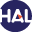# Volume 7 N°2 (2010)

Latest addition : 29 December 2010.

• ### Convergence of Finite Volume MPFA O type Schemes for Heterogeneous Anisotropic Diffusion Problems on General Meshes

29 December 2010,

In this paper we prove the convergence of the finite volume MultiPoint Flux Approximation (MPFA) O scheme for anisotropic and heterogeneous diffusion problems. Our framework is based on a discrete hybrid varia- tional formulation which generalizes the usual construction of the MPFA O scheme. The well-posedness and convergence of the scheme is derived assuming a local coercivity condition which can be easily checked numerically. The novel feature of our framework is that it holds for general polygonal and polyhedral meshes as well as for L∞ diffusion coefficients, which is essential in many practical applications.

Key words : Finite Volume, MPFA, Convergence Analysis, Diffusion Equation, Full Tensor, Anisotropy, Heterogeneities, General Meshes

Paper presented by T. Gallouet on December, 29, 2010Voir sur HAL : https://hal.archives-ouvertes.fr/hal-00340159

• ### Short Notes: Kinetic energy control in explicit Finite Volume discretizations of the incompressible and compressible Navier-Stokes equations

19 November 2010,

Abstract: We prove that, under a cfl condition, the explicit upwind finite volume discretization of the convection operator C(u) = ∂t(ρu) + div(uq), with a given density ρ and momentum q, satisfies a discrete kinetic energy decrease property, provided that the convection operator satisfies a ”consistency-with-the-mass-balance property”, which can be simply stated by saying that it vanishes for a constant advected field u.

Key words : Compressible Navier-Stokes equations, Finite Volume discretizations, Stability, Kinetic Energy.

Paper presented by: Jean-Marc HérardVoir sur HAL : https://hal.archives-ouvertes.fr/hal-01112391

• ### Short Notes: A kinetic energy control in the MAC discretization of compressible Navier-Stokes equations

15 November 2010,

Abstract: We present in this short note a simple construction of the convection operator in the variable density Navier-Stokes equations for MAC discretizations, which ensures the control of the kinetic energy. We thereby extend a similar construction performed in previous works for staggered finite element non-conforming discretizations, and, consequently, the stability results which were obtained for implicit or pressure correction schemes for compressible barotropic flows.

Key words : Compressible Navier-Stokes equations, staggered discretizations, MAC scheme.

Paper presented by: Jean-Marc HérardVoir sur HAL : https://hal.archives-ouvertes.fr/hal-00477079